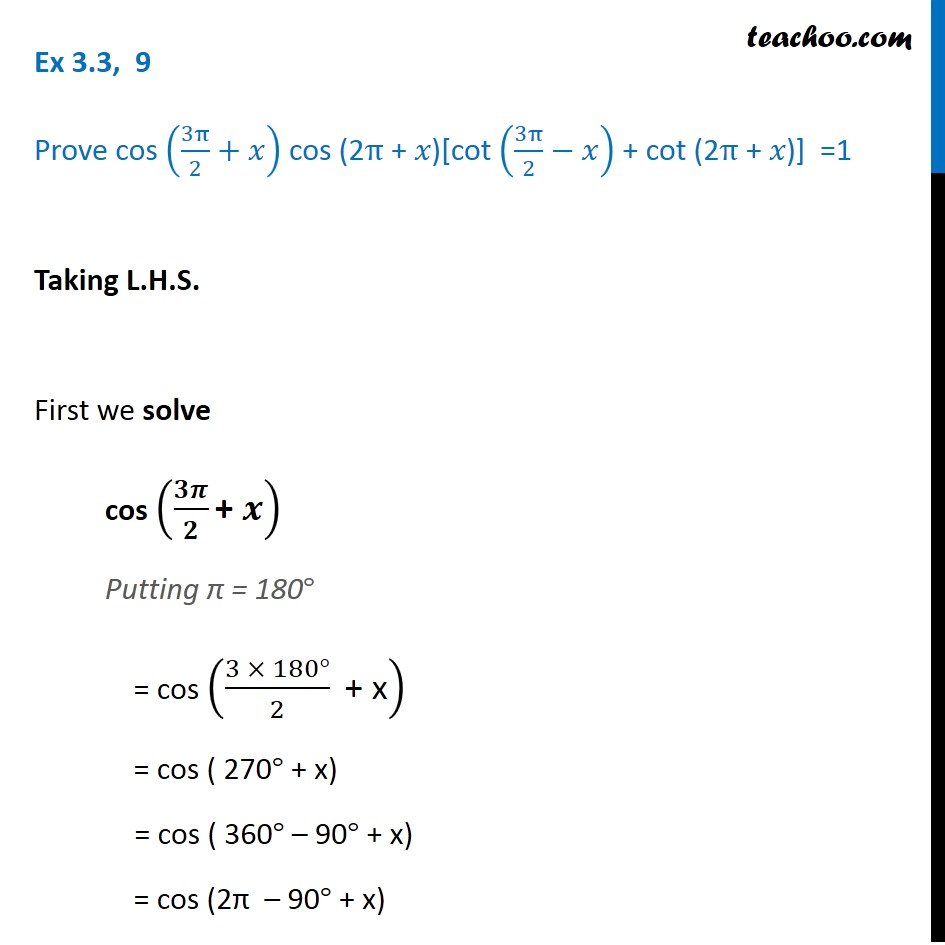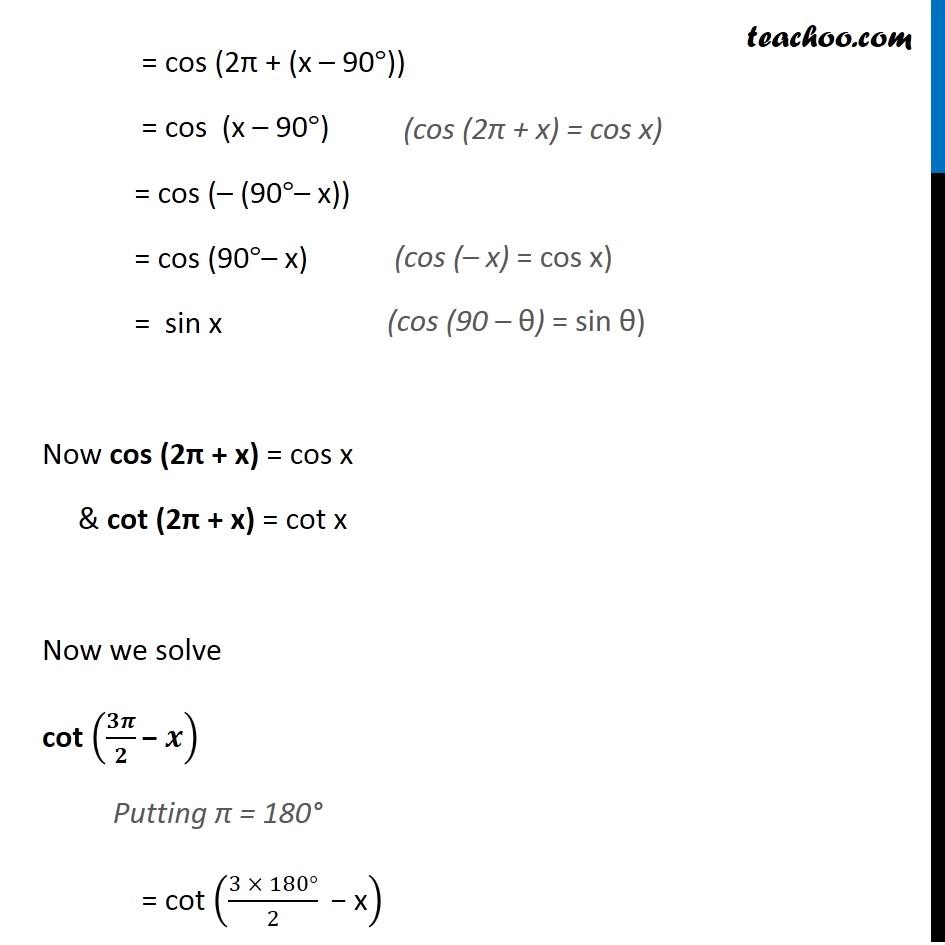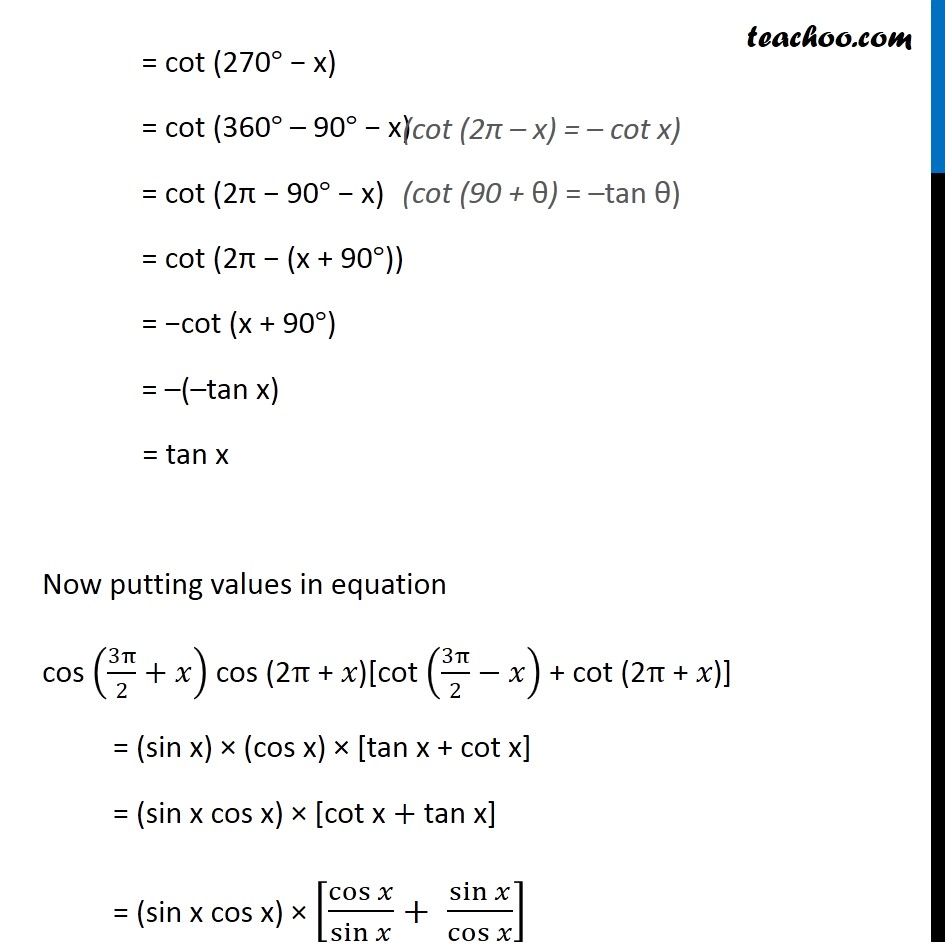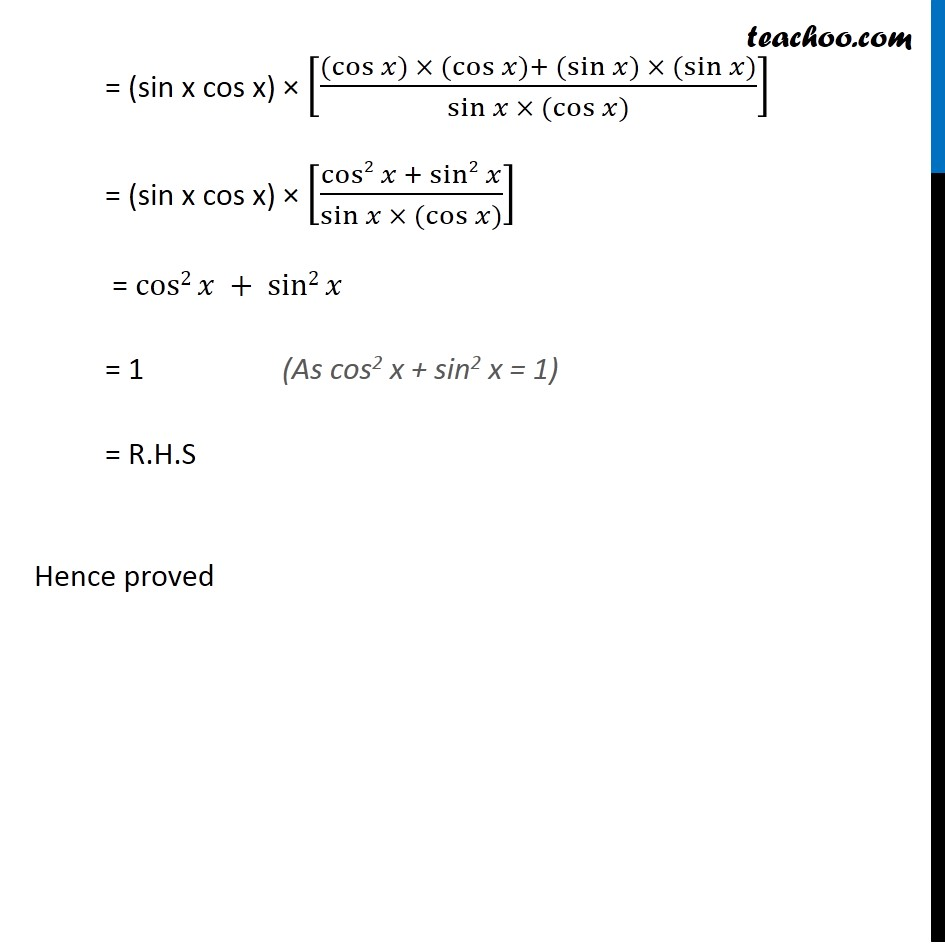Learn All Concepts of Chapter 2 Class 11 Relations and Function - FREE. Check - Trigonometry Class 11 - All Concepts1. Chapter 3 Class 11 Trigonometric Functions
2. Concept wise
3. Finding Value of trignometric functions, given angle

Transcript

Ex 3.3, 9 Prove cos (3π/2+𝑥) cos (2π + 𝑥)[cot (3π/2−𝑥) + cot (2π + 𝑥)] =1 Taking L.H.S. First we solve cos (𝟑𝝅/𝟐 "+ " 𝒙) Putting π = 180° = cos ((3 × 180°)/2 " + x" ) = cos ( 270° + x) = cos ( 360° – 90° + x) = cos (2π – 90° + x) = cos (2π + (x – 90°)) = cos (x – 90°) = cos (– (90°– x)) = cos (90°– x) = sin x Now cos (2π + x) = cos x & cot (2π + x) = cot x Now we solve cot (𝟑𝝅/𝟐 "− " 𝒙) Putting π = 180° = cot ((3 × 180°)/2 " − x" ) = cot (270° "−" x) = cot (360° – 90° "−" x) = cot (2π "−" 90° "−" x) = cot (2π "−" (x + 90°)) = "−"cot (x + 90°) = –(–tan x) = tan x Now putting values in equation cos (3π/2+𝑥) cos (2π + 𝑥)[cot (3π/2−𝑥) + cot (2π + 𝑥)] = (sin x) × (cos x) × [tan x + cot x] = (sin x cos x) × [cot x + tan x] = (sin x cos x) × [cos⁡𝑥/sin⁡𝑥 + sin⁡𝑥/cos⁡𝑥 ] = (sin x cos x) × [(〖(cos〗⁡𝑥) × 〖(cos〗⁡𝑥)+〖 (sin〗⁡𝑥) × 〖(sin〗⁡𝑥))/(sin⁡𝑥 × 〖(cos〗⁡𝑥))] = (sin x cos x) × [(cos2⁡𝑥 +〖 sin2〗⁡𝑥)/(sin⁡𝑥 × 〖(cos〗⁡𝑥))] = cos2⁡𝑥 +〖 sin2〗⁡𝑥 = 1 = R.H.S Hence proved

Finding Value of trignometric functions, given angle

About the AuthorDavneet Singh
Davneet Singh is a graduate from Indian Institute of Technology, Kanpur. He has been teaching from the past 9 years. He provides courses for Maths and Science at Teachoo.